#### Asymptotic stability

Lyapunov stability is weak in that it does not even imply that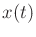converges to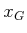as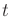approaches infinity. The states are only required to hover around. Convergence requires a stronger notion called asymptotic stability. A pointis an asymptotically stable equilibrium point of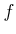if:

1. It is a Lyapunov stable equilibrium point of.
2. There exists some open neighborhood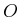ofsuch that, for any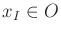,converges15.2 toasapproaches infinity.
For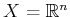, the second condition can be expressed as follows: There exists some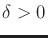such that, for any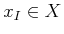with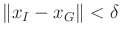, the stateconverges toasapproaches infinity. It may seem strange that two requirements are needed for asymptotic stability. The first one bounds the amount of wiggling room for the integral curve, which is not captured by the second condition.

Asymptotic stability appears to be a reasonable requirement, but it does not imply anything about how long it takes to converge. Ifis asymptotically stable and there exist some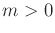and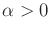such that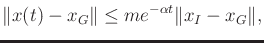(15.2)

thenis also called exponentially stable. This provides a convenient way to express the rate of convergence.

For use in motion planning applications, even exponential convergence may not seem strong enough. This issue was discussed in Section 8.4.1. For example, in practice, one usually prefers to reachin finite time, as opposed to only being reached'' in the limit. There are two common fixes. One is to allow asymptotic stability and declare the goal to be reached if the state arrives in some small, predetermined ball around. In this case, the enlarged goal will always be reached in finite time ifis asymptotically stable. The other fix is to require a stronger form of stability in whichmust be exactly reached in finite time. To enable this, however, discontinuous vector fields such as the inward flow of Figure 8.5b must be used. Most control theorists are appalled by this because infinite energy is usually required to execute such trajectories. On the other hand, discontinuous vector fields may be a suitable representation in some applications, as mentioned in Chapter 8. Note that without feedback this issue does not seem as important. The state trajectories designed in much of Chapter 14 were expected to reach the goal in finite time. Without feedback there was no surrounding vector field that was expected to maintain continuity or smoothness properties. Section 15.1.3 introduces controllability, which is based on actually arriving at the goal in finite time, but it is also based on the existence of one trajectory for a given system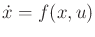, as opposed to a family of trajectories for a given vector field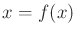.

Steven M LaValle 2020-08-14